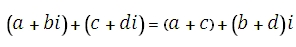# SQL Server IMSUM function

Updated: 27 August 2010

Use IMSUM_q to return the sum of a dataset of complex numbers in x + yi or x + yj text format. The sum of 2 complex numbers is computed as:Syntax
SELECT [wctEngineering].[wct].[IMSUM_q] (
<@IMValues_RangeQuery, nvarchar(4000),>)
Arguments
@IMValues_RangeQuery
the select statement, as text, used to determine the complex numbers to be used in the IMSUM_q calculation.
Return Types
varchar(4000)
Remarks
·         Use COMPLEX to convert real and imaginary coefficients into a complex number.
·         For simpler queries consider using the IMSUM function.
Examples

create
table #s1 (inumber varchar(50))
insert into #s1 values ('2+i')
insert into #s1 values ('2-i')
insert into #s1 values ('3+2i')
insert into #s1 values ('3-2i')

select
wct.imsum_q('select inumber from #s1')

This produces the following result
------------------------
10

(1 row(s) affected)

select

This produces the following result
------------------------
10

(1 row(s) affected)

### SupportCopyright 2008-2021 Westclintech LLC         Privacy Policy        Terms of Service